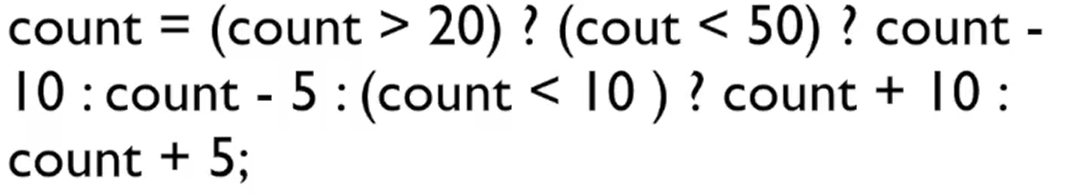## 逻辑运算

``````#include <stdio.h>

int main()
{
int a = -1;
if( a > 0 && a++ > 1 ) {
printf("OK\n");
}
printf("%d\n",a);
return 0;
} ``````
``````-1
Process exited after 0.02138 seconds with return value 0``````

## 条件和逗号运算

``count = (count > 20) ? count - 10 : count + 10 ;``

`````` if(count > 20)
count = count - 10;
else
count = count + 10;``````

::: tip 条件运算符的优先级高于赋值运算符，但是低于其他所有的运算符。 ::: 嵌套条件表达式::: warning 条件运算符是自右向左结合的。 考虑到复杂程度可可读性，要尽量避免使用嵌套条件表达式。 ::: 逗号运算

``````#include <stdio.h>

int main()
{
int i = 0;
i = 2+4,3+5;
printf("%d",i);
return 0;
}``````
``````6
Process exited after 0.01521 seconds with return value 0``````

``````#include <stdio.h>

int main()
{
int i = 0;
i = (2+4,3+5);
printf("%d",i);
return 0;
}``````
``````8
Process exited after 0.01504 seconds with return value 0``````

``for (i = 1 , j = 10 ; i < j ; i++ , j--);``Suzhou
Lv1

17

2

0